# Iterative algorithms for a system of generalized variational inequalities in Hilbert spaces

## Abstract

In this paper, a new system of generalized nonlinear variational inequalities involving three operators is introduced. A three-step iterative algorithm is considered for the system of generalized nonlinear variational inequalities. Strong convergence theorems of the three-step iterative algorithm are established.

MSC:47H05, 47H09, 47J25.

## 1 Introduction

Variational inequalities are among the most interesting and intensively studied classes of mathematical problems and have wide applications in the fields of optimization and control, economics, transportation equilibrium and engineering sciences. There exists a vast amount of literature (see, for instance, ) on the approximation solvability of nonlinear variational inequalities as well as operator equations.

Iterative algorithms have played a central role in the approximation solvability, especially of nonlinear variational inequalities as well as of nonlinear equations, in several fields such as applied mathematics, mathematical programming, mathematical finance, control theory and optimization, engineering sciences and others. Projection methods have played a significant role in the numerical resolution of variational inequalities based on their convergence analysis. However, the convergence analysis does require some sort of strong monotonicity besides the Lipschitz continuity. There have been some recent developments where convergence analysis for projection methods under somewhat weaker conditions such as cocoercivity  and partial relaxed monotonicity  is achieved.

Recently, Chang et al.  introduced a two-step iterative algorithm for a system of nonlinear variational inequalities and established strong convergence theorems. Huang and Noor  introduced the so-called explicit two-step iterative algorithm for a system of nonlinear variational inequalities involving two different nonlinear operators and established strong convergence theorems.

In this paper, we consider, based on the projection method, the approximate solvability of a new system of generalized nonlinear variational inequalities involving three different nonlinear operators in the framework of Hilbert spaces. The results presented in this paper extend and improve the corresponding results announced in Huang and Noor , Chang et al. , Verma  and many others.

Let H be a real Hilbert space, whose inner product and norm are denoted by $〈\cdot ,\cdot 〉$ and $\parallel \cdot \parallel$, respectively. Let C be a nonempty closed convex subset of H and ${P}_{C}$ be the metric projection from H onto C.

Given nonlinear operators $T,f:C\to H$ and $g:C\to C$, we consider the problem of finding $u\in C$ such that

$〈g\left(u\right)-f\left(u\right)+\lambda Tu,v-g\left(u\right)〉\ge 0,\phantom{\rule{1em}{0ex}}\mathrm{\forall }v\in C,$
(1.1)

where $\lambda >0$ is a constant. The variational inequality (1.1) is called the generalized variational inequality involving three operators.

We see that an element $u\in C$ is a solution to the generalized variational inequality (1.1) if and only if $u\in C$ is a fixed point of the mapping

$I-g+{P}_{C}\left(f-\lambda T\right),$

where I is the identity mapping. This equivalence plays an important role in this work.

If $f=g$, then the generalized variational inequality (1.1) is equivalent to the following.

Find $u\in C$ such that

$〈Tu,v-g\left(u\right)〉\ge 0,\phantom{\rule{1em}{0ex}}\mathrm{\forall }v\in C.$
(1.2)

Further, if $g=I$, then the problem (1.2) is reduced to finding $u\in C$ such that

$〈Tu,v-u〉\ge 0,\phantom{\rule{1em}{0ex}}\mathrm{\forall }v\in C,$
(1.3)

which is known as the classical variational inequality originally introduced and studied by Stampacchia .

Let $T:C\to H$ be a mapping. Recall the following definitions.

1. (1)

T is said to be monotone if

$〈Tu-Tv,u-v〉\ge 0,\phantom{\rule{1em}{0ex}}\mathrm{\forall }u,v\in C.$
2. (2)

T is called δ-strongly monotone if there exists a constant $\delta >0$ such that

$〈Tx-Ty,x-y〉\ge \delta {\parallel x-y\parallel }^{2},\phantom{\rule{1em}{0ex}}\mathrm{\forall }x,y\in C.$

This implies that

$\parallel Tx-Ty\parallel \ge \delta \parallel x-y\parallel ,\phantom{\rule{1em}{0ex}}\mathrm{\forall }x,y\in C,$

that is, T is δ-expansive.

1. (3)

T is said to be γ-cocoercive if there exists a constant $\gamma >0$ such that

$〈Tx-Ty,x-y〉\ge \gamma {\parallel Tx-Ty\parallel }^{2},\phantom{\rule{1em}{0ex}}\mathrm{\forall }x,y\in C.$

Clearly, every γ-cocoercive mapping A is $\frac{1}{\gamma }$-Lipschitz continuous.

1. (4)

T is said to be relaxed γ-cocoercive if there exists a constant $\gamma >0$ such that

$〈Tx-Ty,x-y〉\ge \left(-\gamma \right){\parallel Tx-Ty\parallel }^{2},\phantom{\rule{1em}{0ex}}\mathrm{\forall }x,y\in C.$
2. (5)

T is said to be relaxed $\left(\gamma ,\delta \right)$-cocoercive if there exist two constants $\gamma ,\delta >0$ such that

$〈Tx-Ty,x-y〉\ge \left(-\gamma \right){\parallel Tx-Ty\parallel }^{2}+\delta {\parallel x-y\parallel }^{2},\phantom{\rule{1em}{0ex}}\mathrm{\forall }x,y\in C.$

Let ${T}_{i}:C×C×C\to H$, ${f}_{i}:C\to H$ and ${g}_{i}:C\to C$ be nonlinear mappings for each $i=1,2,3$. Consider a system of generalized nonlinear variational inequality (SGNVI) as follows.

Find $\left({x}^{\ast },{y}^{\ast },{z}^{\ast }\right)\in C×C×C$ such that for all $s,t,r>0$,

$\left\{\begin{array}{cc}〈s{T}_{1}\left({y}^{\ast },{z}^{\ast },{x}^{\ast }\right)+{g}_{1}\left({x}^{\ast }\right)-{f}_{1}\left({y}^{\ast }\right),x-{g}_{1}\left({x}^{\ast }\right)〉\ge 0,\hfill & \mathrm{\forall }x\in C,\hfill \\ 〈t{T}_{2}\left({z}^{\ast },{x}^{\ast },{y}^{\ast }\right)+{g}_{2}\left({y}^{\ast }\right)-{f}_{2}\left({z}^{\ast }\right),x-{g}_{2}\left({x}^{\ast }\right)〉\ge 0,\hfill & \mathrm{\forall }x\in C,\hfill \\ 〈r{T}_{3}\left({x}^{\ast },{y}^{\ast },{z}^{\ast }\right)+{g}_{3}\left({z}^{\ast }\right)-{f}_{3}\left({x}^{\ast }\right),x-{g}_{3}\left({x}^{\ast }\right)〉\ge 0,\hfill & \mathrm{\forall }x\in C.\hfill \end{array}$
(1.4)

One can easily see SGNVI (1.4) is equivalent to the following projection problem:

$\left\{\begin{array}{cc}{g}_{1}\left({x}^{\ast }\right)={P}_{C}\left({f}_{1}\left({y}^{\ast }\right)-s{T}_{1}\left({y}^{\ast },{z}^{\ast },{x}^{\ast }\right)\right),\hfill & \mathrm{\forall }s>0,\hfill \\ {g}_{2}\left({y}^{\ast }\right)={P}_{C}\left({f}_{2}\left({z}^{\ast }\right)-t{T}_{2}\left({z}^{\ast },{x}^{\ast },{y}^{\ast }\right)\right),\hfill & \mathrm{\forall }t>0,\hfill \\ {g}_{3}\left({z}^{\ast }\right)={P}_{C}\left({f}_{3}\left({x}^{\ast }\right)-r{T}_{3}\left({x}^{\ast },{y}^{\ast },{z}^{\ast }\right)\right),\hfill & \mathrm{\forall }r>0.\hfill \end{array}$
(1.5)

Next, we consider some special classes of SGNVI (1.4) as follows.

1. (I)

If ${g}_{1}={g}_{2}={g}_{3}=I$, then SGNVI (1.4) is reduced to the following.

Find $\left({x}^{\ast },{y}^{\ast },{z}^{\ast }\right)\in C×C×C$ such that for all $s,t,r>0$,

$\left\{\begin{array}{cc}〈s{T}_{1}\left({y}^{\ast },{z}^{\ast },{x}^{\ast }\right)+{x}^{\ast }-{f}_{1}\left({y}^{\ast }\right),x-{x}^{\ast }〉\ge 0,\hfill & \mathrm{\forall }x\in C,\hfill \\ 〈t{T}_{2}\left({z}^{\ast },{x}^{\ast },{y}^{\ast }\right)+{y}^{\ast }-{f}_{2}\left({z}^{\ast }\right),x-{x}^{\ast }〉\ge 0,\hfill & \mathrm{\forall }x\in C,\hfill \\ 〈r{T}_{3}\left({x}^{\ast },{y}^{\ast },{z}^{\ast }\right)+{z}^{\ast }-{f}_{3}\left({x}^{\ast }\right),x-{x}^{\ast }〉\ge 0,\hfill & \mathrm{\forall }x\in C.\hfill \end{array}$
(1.6)

We see that the problem (1.6) is equivalent to the following projection problem:

$\left\{\begin{array}{cc}{x}^{\ast }={P}_{C}\left({f}_{1}\left({y}^{\ast }\right)-s{T}_{1}\left({y}^{\ast },{z}^{\ast },{x}^{\ast }\right)\right),\hfill & \mathrm{\forall }s>0,\hfill \\ {y}^{\ast }={P}_{C}\left({f}_{2}\left({z}^{\ast }\right)-t{T}_{2}\left({z}^{\ast },{x}^{\ast },{y}^{\ast }\right)\right),\hfill & \mathrm{\forall }t>0,\hfill \\ {z}^{\ast }={P}_{C}\left({f}_{3}\left({x}^{\ast }\right)-r{T}_{3}\left({x}^{\ast },{y}^{\ast },{z}^{\ast }\right)\right),\hfill & \mathrm{\forall }r>0.\hfill \end{array}$
(1.7)
1. (II)

If ${f}_{1}={f}_{2}={f}_{3}=I$, then SGNVI (1.4) is reduced to the following.

Find $\left({x}^{\ast },{y}^{\ast },{z}^{\ast }\right)\in C×C×C$ such that for all $s,t,r>0$,

$\left\{\begin{array}{cc}〈s{T}_{1}\left({y}^{\ast },{z}^{\ast },{x}^{\ast }\right)+{g}_{1}\left({x}^{\ast }\right)-{y}^{\ast },x-{g}_{1}\left({x}^{\ast }\right)〉\ge 0,\hfill & \mathrm{\forall }x\in C,\hfill \\ 〈t{T}_{2}\left({z}^{\ast },{x}^{\ast },{y}^{\ast }\right)+{g}_{2}\left({y}^{\ast }\right)-{z}^{\ast },x-{g}_{2}\left({x}^{\ast }\right)〉\ge 0,\hfill & \mathrm{\forall }x\in C,\hfill \\ 〈r{T}_{3}\left({x}^{\ast },{y}^{\ast },{z}^{\ast }\right)+{g}_{3}\left({z}^{\ast }\right)-{x}^{\ast },x-{g}_{3}\left({x}^{\ast }\right)〉\ge 0,\hfill & \mathrm{\forall }x\in C.\hfill \end{array}$
(1.8)

We see that the problem (1.8) is equivalent to the following projection problem:

$\left\{\begin{array}{cc}{g}_{1}\left({x}^{\ast }\right)={P}_{C}\left[{y}^{\ast }-s{T}_{1}\left({y}^{\ast },{z}^{\ast },{x}^{\ast }\right)\right],\hfill & \mathrm{\forall }s>0,\hfill \\ {g}_{2}\left({y}^{\ast }\right)={P}_{C}\left[{z}^{\ast }-t{T}_{2}\left({z}^{\ast },{x}^{\ast },{y}^{\ast }\right)\right],\hfill & \mathrm{\forall }t>0,\hfill \\ {g}_{3}\left({z}^{\ast }\right)={P}_{C}\left[{x}^{\ast }-r{T}_{3}\left({x}^{\ast },{y}^{\ast },{z}^{\ast }\right)\right],\hfill & \mathrm{\forall }r>0.\hfill \end{array}$
(1.9)
1. (III)

If ${g}_{1}={g}_{2}={g}_{3}={f}_{1}={f}_{2}={f}_{3}=I$, then SGNVI (1.4) is reduced to the following.

Find $\left({x}^{\ast },{y}^{\ast },{z}^{\ast }\right)\in C×C×C$ such that for all $s,t,r>0$,

$\left\{\begin{array}{cc}〈s{T}_{1}\left({y}^{\ast },{z}^{\ast },{x}^{\ast }\right)+{x}^{\ast }-{y}^{\ast },x-{x}^{\ast }〉\ge 0,\hfill & \mathrm{\forall }x\in C,\hfill \\ 〈t{T}_{2}\left({z}^{\ast },{x}^{\ast },{y}^{\ast }\right)+{y}^{\ast }-{z}^{\ast },x-{x}^{\ast }〉\ge 0,\hfill & \mathrm{\forall }x\in C,\hfill \\ 〈r{T}_{3}\left({x}^{\ast },{y}^{\ast },{z}^{\ast }\right)+{z}^{\ast }-{x}^{\ast },x-{x}^{\ast }〉\ge 0,\hfill & \mathrm{\forall }x\in C.\hfill \end{array}$
(1.10)

One can easily get that the problem (1.10) is equivalent to the following projection problem:

$\left\{\begin{array}{cc}{x}^{\ast }={P}_{C}\left({y}^{\ast }-s{T}_{1}\left({y}^{\ast },{z}^{\ast },{x}^{\ast }\right)\right),\hfill & \mathrm{\forall }s>0,\hfill \\ {y}^{\ast }={P}_{C}\left({z}^{\ast }-t{T}_{2}\left({z}^{\ast },{x}^{\ast },{y}^{\ast }\right)\right),\hfill & \mathrm{\forall }t>0,\hfill \\ {z}^{\ast }={P}_{C}\left({x}^{\ast }-r{T}_{3}\left({x}^{\ast },{y}^{\ast },{z}^{\ast }\right)\right),\hfill & \mathrm{\forall }r>0.\hfill \end{array}$
(1.11)
1. (IV)

If ${T}_{1}$, ${T}_{2}$ and ${T}_{3}$ are univariate mappings, then SGNVI (1.4) is reduced to the following.

Find $\left({x}^{\ast },{y}^{\ast },{z}^{\ast }\right)\in C×C×C$ such that for all $s,t,r>0$,

$\left\{\begin{array}{cc}〈s{T}_{1}{y}^{\ast }+{g}_{1}\left({x}^{\ast }\right)-{f}_{1}\left({y}^{\ast }\right),x-{g}_{1}\left({x}^{\ast }\right)〉\ge 0,\hfill & \mathrm{\forall }x\in C,\hfill \\ 〈t{T}_{2}{z}^{\ast }+{g}_{2}\left({y}^{\ast }\right)-{f}_{2}\left({z}^{\ast }\right),x-{g}_{2}\left({x}^{\ast }\right)〉\ge 0,\hfill & \mathrm{\forall }x\in C,\hfill \\ 〈r{T}_{3}{x}^{\ast }+{g}_{3}\left({z}^{\ast }\right)-{f}_{3}\left({x}^{\ast }\right),x-{g}_{3}\left({x}^{\ast }\right)〉\ge 0,\hfill & \mathrm{\forall }x\in C.\hfill \end{array}$
(1.12)

One can easily see that the problem (1.12) is equivalent to the following projection problem:

$\left\{\begin{array}{cc}{g}_{1}\left({x}^{\ast }\right)={P}_{C}\left({f}_{1}\left({y}^{\ast }\right)-s{T}_{1}{y}^{\ast }\right),\hfill & \mathrm{\forall }s>0,\hfill \\ {g}_{2}\left({y}^{\ast }\right)={P}_{C}\left({f}_{2}\left({z}^{\ast }\right)-t{T}_{2}{z}^{\ast }\right),\hfill & \mathrm{\forall }t>0,\hfill \\ {g}_{3}\left({z}^{\ast }\right)={P}_{C}\left({f}_{3}\left({x}^{\ast }\right)-r{T}_{3}{x}^{\ast }\right),\hfill & \mathrm{\forall }r>0.\hfill \end{array}$
(1.13)

## 2 Preliminaries

In this section, to study the approximate solvability of the problems (1.4), (1.6), (1.8), (1.10) and (1.12), we introduce the following three-step algorithms.

Algorithm 2.1 For any $\left({x}_{0},{y}_{0},{z}_{0}\right)\in C×C×C$, compute the sequences $\left\{{x}_{n}\right\}$, $\left\{{y}_{n}\right\}$ and $\left\{{z}_{n}\right\}$ by the following iterative process:

$\left\{\begin{array}{cc}{x}_{n+1}=\left(1-{\alpha }_{n}\right){x}_{n}+{\alpha }_{n}\left({x}_{n}-{g}_{1}\left({x}_{n}\right)+{P}_{C}\left({f}_{1}\left({y}_{n}\right)-s{T}_{1}\left({y}_{n},{z}_{n},{x}_{n}\right)\right)\right),\hfill & n\ge 0,\hfill \\ {g}_{3}\left({z}_{n+1}\right)={P}_{C}\left({f}_{3}\left({x}_{n+1}\right)-r{T}_{3}\left({x}_{n+1},{y}_{n},{z}_{n}\right)\right),\hfill & n\ge 0,\hfill \\ {g}_{2}\left({y}_{n+1}\right)={P}_{C}\left({f}_{2}\left({z}_{n+1}\right)-t{T}_{2}\left({z}_{n+1},{x}_{n},{y}_{n}\right)\right),\hfill & n\ge 0,\hfill \end{array}$

where $r,s,t>0$ are three constants and $\left\{{\alpha }_{n}\right\}$ is a sequence in $\left[0,1\right]$.

If ${g}_{1}={g}_{2}={g}_{3}=I$, then Algorithm 2.1 is reduced to the following.

Algorithm 2.2 For any $\left({x}_{0},{y}_{0},{z}_{0}\right)\in C×C×C$, compute the sequences $\left\{{x}_{n}\right\}$, $\left\{{y}_{n}\right\}$ and $\left\{{z}_{n}\right\}$ by the following iterative process:

$\left\{\begin{array}{cc}{x}_{n+1}=\left(1-{\alpha }_{n}\right){x}_{n}+{\alpha }_{n}{P}_{C}\left({f}_{1}\left({y}_{n}\right)-s{T}_{1}\left({y}_{n},{z}_{n},{x}_{n}\right)\right),\hfill & n\ge 0,\hfill \\ {z}_{n+1}={P}_{C}\left({f}_{3}\left({x}_{n+1}\right)-r{T}_{3}\left({x}_{n+1},{y}_{n},{z}_{n}\right)\right),\hfill & n\ge 0,\hfill \\ {y}_{n+1}={P}_{C}\left({f}_{2}\left({z}_{n+1}\right)-t{T}_{2}\left({z}_{n+1},{x}_{n},{y}_{n}\right)\right),\hfill & n\ge 0,\hfill \end{array}$

where $r,s,t>0$ are three constants and $\left\{{\alpha }_{n}\right\}$ is a sequence in $\left[0,1\right]$.

If ${f}_{1}={f}_{2}={f}_{3}=I$, the identity mapping, then Algorithm 2.1 is reduced to the following.

Algorithm 2.3 For any $\left({x}_{0},{y}_{0},{z}_{0}\right)\in C×C×C$, compute the sequences $\left\{{x}_{n}\right\}$, $\left\{{y}_{n}\right\}$ and $\left\{{z}_{n}\right\}$ by the following iterative process:

$\left\{\begin{array}{cc}{x}_{n+1}=\left(1-{\alpha }_{n}\right){x}_{n}+{\alpha }_{n}\left({x}_{n}-{g}_{1}\left({x}_{n}\right)+{P}_{C}\left({y}_{n}-s{T}_{1}\left({y}_{n},{z}_{n},{x}_{n}\right)\right)\right),\hfill & n\ge 0,\hfill \\ {g}_{3}\left({z}_{n+1}\right)={P}_{C}\left({x}_{n+1}-r{T}_{3}\left({x}_{n+1},{y}_{n},{z}_{n}\right)\right),\hfill & n\ge 0,\hfill \\ {g}_{2}\left({y}_{n+1}\right)={P}_{C}\left({z}_{n+1}-t{T}_{2}\left({z}_{n+1},{x}_{n},{y}_{n}\right)\right),\hfill & n\ge 0,\hfill \end{array}$

where $r,s,t>0$ are three constants and $\left\{{\alpha }_{n}\right\}$ is a sequence in $\left[0,1\right]$.

If ${f}_{1}={f}_{2}={f}_{3}={g}_{1}={g}_{2}={g}_{3}=I$, the identity mapping, then Algorithm 2.1 is reduced to the following.

Algorithm 2.4 For any $\left({x}_{0},{y}_{0},{z}_{0}\right)\in C×C×C$, compute the sequences $\left\{{x}_{n}\right\}$, $\left\{{y}_{n}\right\}$ and $\left\{{z}_{n}\right\}$ by the following iterative process:

$\left\{\begin{array}{cc}{x}_{n+1}=\left(1-{\alpha }_{n}\right){x}_{n}+{\alpha }_{n}{P}_{C}\left({y}_{n}-s{T}_{1}\left({y}_{n},{z}_{n},{x}_{n}\right)\right),\hfill & n\ge 0,\hfill \\ {z}_{n+1}={P}_{C}\left({x}_{n+1}-r{T}_{3}\left({x}_{n+1},{y}_{n},{z}_{n}\right)\right),\hfill & n\ge 0,\hfill \\ {y}_{n+1}={P}_{C}\left({z}_{n+1}-t{T}_{2}\left({z}_{n+1},{x}_{n},{y}_{n}\right)\right),\hfill & n\ge 0,\hfill \end{array}$

where $r,s,t>0$ are three constants and $\left\{{\alpha }_{n}\right\}$ is a sequence in $\left[0,1\right]$.

1. (IV)

If ${T}_{1}$, ${T}_{2}$ and ${T}_{3}$ are univariate mappings, then Algorithm 2.1 is reduced to the following.

Algorithm 2.5 For any $\left({x}_{0},{y}_{0},{z}_{0}\right)\in C×C×C$, compute the sequences $\left\{{x}_{n}\right\}$, $\left\{{y}_{n}\right\}$ and $\left\{{z}_{n}\right\}$ by the following iterative process:

$\left\{\begin{array}{cc}{x}_{n+1}=\left(1-{\alpha }_{n}\right){x}_{n}+{\alpha }_{n}\left({x}_{n}-{g}_{1}\left({x}_{n}\right)+{P}_{C}\left({f}_{1}\left({y}_{n}\right)-s{T}_{1}{y}_{n}\right)\right),\hfill & n\ge 0,\hfill \\ {g}_{3}\left({z}_{n+1}\right)={P}_{C}\left({f}_{3}\left({x}_{n+1}\right)-r{T}_{3}{x}_{n+1}\right),\hfill & n\ge 0,\hfill \\ {g}_{2}\left({y}_{n+1}\right)={P}_{C}\left({f}_{2}\left({z}_{n+1}\right)-t{T}_{2}{z}_{n+1}\right),\hfill & n\ge 0,\hfill \end{array}$

where $r,s,t>0$ are three constants and $\left\{{\alpha }_{n}\right\}$ is a sequence in $\left[0,1\right]$.

In order to prove our main results, we also need the following lemma and definitions.

Lemma 2.6 

Assume that $\left\{{a}_{n}\right\}$ is a sequence of nonnegative real numbers such that

${a}_{n+1}\le \left(1-{\lambda }_{n}\right){a}_{n}+{b}_{n},\phantom{\rule{1em}{0ex}}\mathrm{\forall }n\ge {n}_{0},$

where ${n}_{0}$ is a nonnegative integer, $\left\{{\lambda }_{n}\right\}$ is a sequence in $\left(0,1\right)$ with ${\sum }_{n=1}^{\mathrm{\infty }}{\lambda }_{n}=\mathrm{\infty }$ and ${b}_{n}=\circ \left({\lambda }_{n}\right)$, then ${lim}_{n\to \mathrm{\infty }}{a}_{n}=0$.

Definition 2.7 A mapping $T:C×C×C\to H$ is said to be relaxed $\left(\gamma ,\delta \right)$-cocoercive if there exist constants $\gamma ,\delta >0$ such that for all $x,{x}^{\prime }\in C$,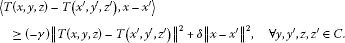Definition 2.8 A mapping $T:C×C×C\to H$ is said to be β-Lipschitz continuous in the first variable if there exists a constant $\beta >0$ such that for all $x,{x}^{\prime }\in C$,

$\parallel T\left(x,y,z\right)-T\left({x}^{\prime },{y}^{\prime },{z}^{\prime }\right)\parallel \le \beta \parallel x-{x}^{\prime }\parallel ,\phantom{\rule{1em}{0ex}}\mathrm{\forall }y,{y}^{\prime },z,{z}^{\prime }\in C.$

## 3 Main results

Theorem 3.1 Let C be a nonempty, closed and convex subset of a real Hilbert space H. Let ${T}_{i}:C×C×C\to H$ be a relaxed $\left({\gamma }_{i},{\delta }_{i}\right)$-cocoercive and ${\beta }_{i}$-Lipschitz continuous mapping in the first variable, ${f}_{i}:C\to H$ be a relaxed $\left({\eta }_{i},{\rho }_{i}\right)$-cocoercive and ${\lambda }_{i}$-Lipschitz continuous mapping and ${g}_{i}:C\to C$ be a relaxed $\left({\overline{\eta }}_{i},{\overline{\rho }}_{i}\right)$-cocoercive and ${\overline{\lambda }}_{i}$-Lipschitz continuous mapping for each $i=1,2,3$. Suppose that $\left({x}^{\ast },{y}^{\ast },{z}^{\ast }\right)\in C×C×C$ is a solution to the problem (1.4). Let $\left\{{x}_{n}\right\}$, $\left\{{y}_{n}\right\}$ and $\left\{{z}_{n}\right\}$ be the sequences generated by Algorithm 2.1. Assume that the following conditions are satisfied:

1. (a)

${\sum }_{n=0}^{\mathrm{\infty }}{\alpha }_{n}=\mathrm{\infty }$;

2. (b)

$0\le {\theta }_{6}$, ${\theta }_{9}<1$;

3. (c)

$\left({\theta }_{1}+{\theta }_{2}\right)\left({\theta }_{4}+{\theta }_{5}\right)\left({\theta }_{7}+{\theta }_{8}\right)\le \left(1-{\theta }_{3}\right)\left(1-{\theta }_{6}\right)\left(1-{\theta }_{9}\right)$,

where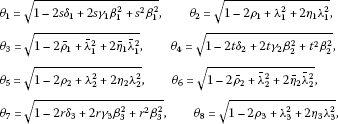and

${\theta }_{9}=\sqrt{1-2{\overline{\rho }}_{3}+{\overline{\lambda }}_{3}^{2}+2{\overline{\eta }}_{3}{\overline{\lambda }}_{3}^{2}}.$

Then the sequences $\left\{{x}_{n}\right\}$, $\left\{{y}_{n}\right\}$ and $\left\{{z}_{n}\right\}$ converge strongly to ${x}^{\ast }$, ${y}^{\ast }$ and ${z}^{\ast }$, respectively.

Proof In view of $\left({x}^{\ast },{y}^{\ast },{z}^{\ast }\right)$ being a solution to the problem (1.4), we see that

$\left\{\begin{array}{cc}{x}^{\ast }=\left(1-{\alpha }_{n}\right){x}^{\ast }+{\alpha }_{n}\left({x}^{\ast }-{g}_{1}\left({x}^{\ast }\right)+{P}_{C}\left({f}_{1}\left({y}^{\ast }\right)-s{T}_{1}\left({y}^{\ast },{z}^{\ast },{x}^{\ast }\right)\right)\right),\hfill & n\ge 0,\hfill \\ {g}_{3}\left({z}^{\ast }\right)={P}_{C}\left({f}_{3}\left({x}^{\ast }\right)-r{T}_{3}\left({x}^{\ast },{y}^{\ast },{z}^{\ast }\right)\right),\hfill & n\ge 0,\hfill \\ {g}_{2}\left({y}^{\ast }\right)={P}_{C}\left({f}_{2}\left({z}^{\ast }\right)-t{T}_{2}\left({z}^{\ast },{x}^{\ast },{y}^{\ast }\right)\right),\hfill & n\ge 0.\hfill \end{array}$

It follows from Algorithm (2.1) that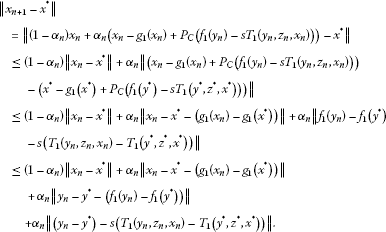(3.1)

By the assumption that ${T}_{1}$ is relaxed $\left({\gamma }_{1},{r}_{1}\right)$-cocoercive and ${\beta }_{1}$-Lipschitz continuous in the first variable, we obtain that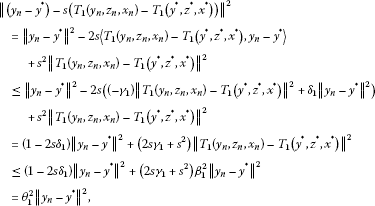(3.2)

where ${\theta }_{1}=\sqrt{1-2s{\delta }_{1}+2s{\gamma }_{1}{\beta }_{1}^{2}+{s}^{2}{\beta }_{1}^{2}}$. On the other hand, it follows from the assumption that ${f}_{1}$ is relaxed $\left({\eta }_{1},{\rho }_{1}\right)$-cocoercive and ${\lambda }_{1}$-Lipschitz continuous that(3.3)

where ${\theta }_{2}=\sqrt{1-2{\rho }_{1}+{\lambda }_{1}^{2}+2{\eta }_{1}{\lambda }_{1}^{2}}$. In a similar way, we can obtain that

$\parallel {x}_{n}-{x}^{\ast }-\left({g}_{1}\left({x}_{n}\right)-{g}_{1}\left({x}^{\ast }\right)\right)\parallel \le {\theta }_{3}\parallel {x}_{n}-{x}^{\ast }\parallel ,$
(3.4)

where ${\theta }_{3}=\sqrt{1-2{\overline{\rho }}_{1}+{\overline{\lambda }}_{1}^{2}+2{\overline{\eta }}_{1}{\overline{\lambda }}_{1}^{2}}$. Substituting (3.2), (3.3) and (3.4) into (3.1), we arrive at

$\parallel {x}_{n+1}-{x}^{\ast }\parallel \le \left[1-{\alpha }_{n}\left(1-{\theta }_{3}\right)\right]\parallel {x}_{n}-{x}^{\ast }\parallel +{\alpha }_{n}\left({\theta }_{1}+{\theta }_{2}\right)\parallel {y}_{n}-{y}^{\ast }\parallel .$
(3.5)

Next, we estimate $\parallel {y}_{n}-{y}^{\ast }\parallel$. From Algorithm 2.1, we see that

$\begin{array}{rcl}\parallel {g}_{2}\left({y}_{n+1}\right)-{g}_{2}\left({y}^{\ast }\right)\parallel & =& \parallel {P}_{C}\left({f}_{2}\left({z}_{n+1}\right)-t{T}_{2}\left({z}_{n+1},{x}_{n},{y}_{n}\right)\right)\\ -{P}_{C}\left({f}_{2}\left({z}^{\ast }\right)-t{T}_{2}\left({z}^{\ast },{x}^{\ast },{y}^{\ast }\right)\right)\parallel \\ \le & \parallel \left({f}_{2}\left({z}_{n+1}\right)-t{T}_{2}\left({z}_{n+1},{x}_{n},{y}_{n}\right)\right)-\left({f}_{2}\left({z}^{\ast }\right)-t{T}_{2}\left({z}^{\ast },{x}^{\ast },{y}^{\ast }\right)\right)\parallel \\ \le & \parallel \left({z}_{n+1}-{z}^{\ast }\right)-\left({f}_{2}\left({z}_{n+1}\right)-{f}_{2}\left({z}^{\ast }\right)\right)\parallel \\ +\parallel \left({z}_{n+1}-{z}^{\ast }\right)-t\left({T}_{2}\left({z}_{n+1},{x}_{n},{y}_{n}\right)-{T}_{2}\left({z}^{\ast },{x}^{\ast },{y}^{\ast }\right)\right)\parallel .\end{array}$
(3.6)

By the assumption that ${T}_{2}$ is relaxed $\left({\gamma }_{2},{r}_{2}\right)$-cocoercive and ${\beta }_{2}$-Lipschitz continuous in the first variable, we obtain that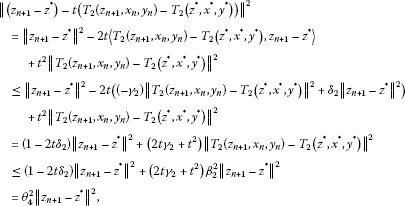(3.7)

where ${\theta }_{4}=\sqrt{1-2t{\delta }_{2}+2t{\gamma }_{2}{\beta }_{2}^{2}+{t}^{2}{\beta }_{2}^{2}}$. It follows from the assumption that ${f}_{2}$ is relaxed $\left({\eta }_{2},{\rho }_{2}\right)$-cocoercive and ${\lambda }_{2}$-Lipschitz continuous that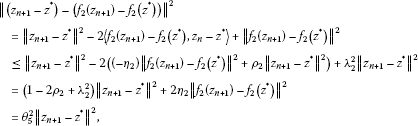(3.8)

where ${\theta }_{5}=\sqrt{1-2{\rho }_{2}+{\lambda }_{2}^{2}+2{\eta }_{2}{\lambda }_{2}^{2}}$. Substituting (3.7) and (3.8) into (3.6), we see that

$\parallel {g}_{2}\left({y}_{n+1}\right)-{g}_{2}\left({y}^{\ast }\right)\parallel \le \left({\theta }_{4}+{\theta }_{5}\right)\parallel {z}_{n+1}-{z}^{\ast }\parallel .$
(3.9)

On the other hand, we have

$\parallel {y}_{n+1}-{y}^{\ast }\parallel \le \parallel {y}_{n+1}-{y}^{\ast }-\left({g}_{2}\left({y}_{n+1}\right)-{g}_{2}\left({y}^{\ast }\right)\right)\parallel +\parallel {g}_{2}\left({y}_{n+1}\right)-{g}_{2}\left({y}^{\ast }\right)\parallel .$
(3.10)

From the proof of (3.8), we arrive at

$\parallel \left({y}_{n+1}-{y}^{\ast }\right)-\left({g}_{2}\left({y}_{n+1}\right)-{g}_{2}\left({y}^{\ast }\right)\right)\parallel \le {\theta }_{6}\parallel {y}_{n+1}-{y}^{\ast }\parallel ,$
(3.11)

where ${\theta }_{6}=\sqrt{1-2{\overline{\rho }}_{2}+{\overline{\lambda }}_{2}^{2}+2{\overline{\eta }}_{2}{\overline{\lambda }}_{2}^{2}}$. Substituting (3.9) and (3.11) into (3.10), we see that

$\parallel {y}_{n+1}-{y}^{\ast }\parallel \le {\theta }_{6}\parallel {y}_{n+1}-{y}^{\ast }\parallel +\left({\theta }_{4}+{\theta }_{5}\right)\parallel {z}_{n+1}-{z}^{\ast }\parallel .$

It follows from the condition (b) that

$\parallel {y}_{n+1}-{y}^{\ast }\parallel \le \frac{{\theta }_{4}+{\theta }_{5}}{1-{\theta }_{6}}\parallel {z}_{n+1}-{z}^{\ast }\parallel .$

That is,

$\parallel {y}_{n}-{y}^{\ast }\parallel \le \frac{{\theta }_{4}+{\theta }_{5}}{1-{\theta }_{6}}\parallel {z}_{n}-{z}^{\ast }\parallel .$
(3.12)

Finally, we estimate $\parallel {z}_{n}-{z}^{\ast }\parallel$. It follows from Algorithm 2.1 that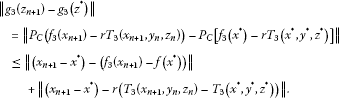(3.13)

In a similar way, we can show that

$\parallel \left({x}_{n+1}-{x}^{\ast }\right)-r\left({T}_{3}\left({x}_{n+1},{y}_{n},{z}_{n}\right)-{T}_{3}\left({x}^{\ast },{y}^{\ast },{z}^{\ast }\right)\right)\parallel \le {\theta }_{7}\parallel {x}_{n+1}-{x}^{\ast }\parallel$
(3.14)

and

$\parallel \left({x}_{n+1}-{x}^{\ast }\right)-\left({f}_{3}\left({x}_{n+1}\right)-{f}_{3}\left({x}^{\ast }\right)\right)\parallel \le {\theta }_{8}\parallel {x}_{n+1}-{x}^{\ast }\parallel ,$
(3.15)

where ${\theta }_{7}=\sqrt{1-2r{\delta }_{3}+2r{\gamma }_{3}{\beta }_{3}^{2}+{r}^{2}{\beta }_{3}^{2}}$ and ${\theta }_{8}=\sqrt{1-2{\rho }_{3}+{\lambda }_{3}^{2}+2{\eta }_{3}{\lambda }_{3}^{2}}$. Substituting (3.14) and (3.15) into (3.13), we arrive at

$\parallel {g}_{3}\left({z}_{n+1}\right)-{g}_{3}\left({z}^{\ast }\right)\parallel \le \left({\theta }_{7}+{\theta }_{8}\right)\parallel {x}_{n+1}-{x}^{\ast }\parallel .$
(3.16)

Note that

$\parallel {z}_{n+1}-{z}^{\ast }\parallel \le \parallel {z}_{n+1}-{z}^{\ast }-\left({g}_{3}\left({z}_{n+1}\right)-{g}_{3}\left({z}^{\ast }\right)\right)\parallel +\parallel {g}_{3}\left({z}_{n+1}\right)-{g}_{3}\left({z}^{\ast }\right)\parallel .$
(3.17)

On the other hand, we have

$\parallel {z}_{n+1}-{z}^{\ast }-\left({g}_{3}\left({z}_{n+1}\right)-{g}_{3}\left({z}^{\ast }\right)\right)\parallel \le {\theta }_{9}\parallel {z}_{n+1}-{z}^{\ast }\parallel ,$
(3.18)

${\theta }_{9}=\sqrt{1-2{\overline{\rho }}_{3}+{\overline{\lambda }}_{3}^{2}+2{\overline{\eta }}_{3}{\overline{\lambda }}_{3}^{2}}$. Substituting (3.16) and (3.18) into (3.17), we arrive at

$\parallel {z}_{n+1}-{z}^{\ast }\parallel \le {\theta }_{9}\parallel {z}_{n+1}-{z}^{\ast }\parallel +\left({\theta }_{7}+{\theta }_{8}\right)\parallel {x}_{n+1}-{x}^{\ast }\parallel .$

It follows from the condition (b) that

$\parallel {z}_{n+1}-{z}^{\ast }\parallel \le \frac{{\theta }_{7}+{\theta }_{8}}{1-{\theta }_{9}}\parallel {x}_{n+1}-{x}^{\ast }\parallel .$

That is,

$\parallel {z}_{n}-{z}^{\ast }\parallel \le \frac{{\theta }_{7}+{\theta }_{8}}{1-{\theta }_{9}}\parallel {x}_{n}-{x}^{\ast }\parallel .$
(3.19)

Combining (3.5), (3.12) with (3.19), we obtain that

$\parallel {x}_{n+1}-{x}^{\ast }\parallel \le \left(1-{\alpha }_{n}\left(1-{\theta }_{3}-\left({\theta }_{1}+{\theta }_{2}\right)\frac{{\theta }_{4}+{\theta }_{5}}{1-{\theta }_{6}}\frac{{\theta }_{7}+{\theta }_{8}}{1-{\theta }_{9}}\right)\right)\parallel {x}_{n}-{x}^{\ast }\parallel .$

Since ${\sum }_{n=0}^{\mathrm{\infty }}{\alpha }_{n}=\mathrm{\infty }$ and the condition (c), we can conclude the desired conclusion easily from Lemma 2.6. This completes the proof. □

Remark 3.2 Theorem 3.1 includes the corresponding results in Huang and Noor  Chang et al. , and Verma  as special cases.

From Theorem 3.1, we can get the following results immediately.

Corollary 3.3 Let C be a nonempty, closed and convex subset of a real Hilbert space H. Let ${T}_{i}:C×C×C\to H$ be a relaxed $\left({\gamma }_{i},{\delta }_{i}\right)$-cocoercive and ${\beta }_{i}$-Lipschitz continuous mapping in the first variable and ${f}_{i}:C\to H$ be a relaxed $\left({\eta }_{i},{\rho }_{i}\right)$-cocoercive and ${\lambda }_{i}$-Lipschitz continuous mapping for each $i=1,2,3$. Suppose that $\left({x}^{\ast },{y}^{\ast },{z}^{\ast }\right)\in C×C×C$ is a solution to the problem (1.6). Let $\left\{{x}_{n}\right\}$, $\left\{{y}_{n}\right\}$ and $\left\{{z}_{n}\right\}$ be the sequences generated by Algorithm 2.2. Assume that the following conditions are satisfied:

1. (a)

${\sum }_{n=0}^{\mathrm{\infty }}{\alpha }_{n}=\mathrm{\infty }$;

2. (b)

$\left({\theta }_{1}+{\theta }_{2}\right)\left({\theta }_{4}+{\theta }_{5}\right)\left({\theta }_{7}+{\theta }_{8}\right)\le 1$,

whereand

${\theta }_{7}=\sqrt{1-2r{\delta }_{3}+2r{\gamma }_{3}{\beta }_{3}^{2}+{r}^{2}{\beta }_{3}^{2}},\phantom{\rule{2em}{0ex}}{\theta }_{8}=\sqrt{1-2{\rho }_{3}+{\lambda }_{3}^{2}+2{\eta }_{3}{\lambda }_{3}^{2}}.$

Then the sequences $\left\{{x}_{n}\right\}$, $\left\{{y}_{n}\right\}$ and $\left\{{z}_{n}\right\}$ converge strongly to ${x}^{\ast }$, ${y}^{\ast }$ and ${z}^{\ast }$, respectively.

Corollary 3.4 Let C be a nonempty, closed and convex subset of a real Hilbert space H. Let ${T}_{i}:C×C×C\to H$ be a relaxed $\left({\gamma }_{i},{\delta }_{i}\right)$-cocoercive and ${\beta }_{i}$-Lipschitz continuous mapping in the first variable and ${g}_{i}:C\to C$ be a relaxed $\left({\overline{\eta }}_{i},{\overline{\rho }}_{i}\right)$-cocoercive and ${\overline{\lambda }}_{i}$-Lipschitz continuous mapping for each $i=1,2,3$. Suppose that $\left({x}^{\ast },{y}^{\ast },{z}^{\ast }\right)\in C×C×C$ is a solution to the problem (1.8). Let $\left\{{x}_{n}\right\}$, $\left\{{y}_{n}\right\}$ and $\left\{{z}_{n}\right\}$ be the sequences generated by Algorithm 2.3. Assume that the following conditions are satisfied:

1. (a)

${\sum }_{n=0}^{\mathrm{\infty }}{\alpha }_{n}=\mathrm{\infty }$;

2. (b)

$0\le {\theta }_{6}$, ${\theta }_{9}<1$;

3. (c)

${\theta }_{1}{\theta }_{4}{\theta }_{7}\le \left(1-{\theta }_{3}\right)\left(1-{\theta }_{6}\right)\left(1-{\theta }_{9}\right)$,

where

$\begin{array}{r}{\theta }_{1}=\sqrt{1-2s{\delta }_{1}+2s{\gamma }_{1}{\beta }_{1}^{2}+{s}^{2}{\beta }_{1}^{2}},\phantom{\rule{2em}{0ex}}{\theta }_{3}=\sqrt{1-2{\overline{\rho }}_{1}+{\overline{\lambda }}_{1}^{2}+2{\overline{\eta }}_{1}{\overline{\lambda }}_{1}^{2}},\\ {\theta }_{4}=\sqrt{1-2t{\delta }_{2}+2t{\gamma }_{2}{\beta }_{2}^{2}+{t}^{2}{\beta }_{2}^{2}},\phantom{\rule{2em}{0ex}}{\theta }_{6}=\sqrt{1-2{\overline{\rho }}_{2}+{\overline{\lambda }}_{2}^{2}+2{\overline{\eta }}_{2}{\overline{\lambda }}_{2}^{2}},\end{array}$

and

${\theta }_{7}=\sqrt{1-2r{\delta }_{3}+2r{\gamma }_{3}{\beta }_{3}^{2}+{r}^{2}{\beta }_{3}^{2}},\phantom{\rule{2em}{0ex}}{\theta }_{9}=\sqrt{1-2{\overline{\rho }}_{3}+{\overline{\lambda }}_{3}^{2}+2{\overline{\eta }}_{3}{\overline{\lambda }}_{3}^{2}}.$

Then the sequences $\left\{{x}_{n}\right\}$, $\left\{{y}_{n}\right\}$ and $\left\{{z}_{n}\right\}$ converge strongly to ${x}^{\ast }$, ${y}^{\ast }$ and ${z}^{\ast }$, respectively.

Corollary 3.5 Let C be a nonempty, closed and convex subset of a real Hilbert space H. Let ${T}_{i}:C×C×C\to H$ be a relaxed $\left({\gamma }_{i},{\delta }_{i}\right)$-cocoercive and ${\beta }_{i}$-Lipschitz continuous mapping in the first variable for each $i=1,2,3$. Suppose that $\left({x}^{\ast },{y}^{\ast },{z}^{\ast }\right)\in C×C×C$ is a solution to the problem (1.10). Let $\left\{{x}_{n}\right\}$, $\left\{{y}_{n}\right\}$ and $\left\{{z}_{n}\right\}$ be the sequences generated by Algorithm 2.4. Assume that the following conditions are satisfied:

1. (a)

${\sum }_{n=0}^{\mathrm{\infty }}{\alpha }_{n}=\mathrm{\infty }$;

2. (b)

${\theta }_{1}{\theta }_{4}{\theta }_{7}\le 1$,

where

${\theta }_{1}=\sqrt{1-2s{\delta }_{1}+2s{\gamma }_{1}{\beta }_{1}^{2}+{s}^{2}{\beta }_{1}^{2}},\phantom{\rule{2em}{0ex}}{\theta }_{4}=\sqrt{1-2t{\delta }_{2}+2t{\gamma }_{2}{\beta }_{2}^{2}+{t}^{2}{\beta }_{2}^{2}},$

and

${\theta }_{7}=\sqrt{1-2r{\delta }_{3}+2r{\gamma }_{3}{\beta }_{3}^{2}+{r}^{2}{\beta }_{3}^{2}}.$

Then the sequences $\left\{{x}_{n}\right\}$, $\left\{{y}_{n}\right\}$ and $\left\{{z}_{n}\right\}$ converge strongly to ${x}^{\ast }$, ${y}^{\ast }$ and ${z}^{\ast }$, respectively.

Corollary 3.6 Let C be a nonempty, closed and convex subset of a real Hilbert space H. Let ${T}_{i}:C\to H$ be a relaxed $\left({\gamma }_{i},{\delta }_{i}\right)$-cocoercive and ${\beta }_{i}$-Lipschitz continuous mapping, ${f}_{i}:C\to H$ be a relaxed $\left({\eta }_{i},{\rho }_{i}\right)$-cocoercive and ${\lambda }_{i}$-Lipschitz continuous mapping and ${g}_{i}:C\to C$ be a relaxed $\left({\overline{\eta }}_{i},{\overline{\rho }}_{i}\right)$-cocoercive and ${\overline{\lambda }}_{i}$-Lipschitz continuous mapping for each $i=1,2,3$. Suppose that $\left({x}^{\ast },{y}^{\ast },{z}^{\ast }\right)\in C×C×C$ is a solution to the problem (1.12). Let $\left\{{x}_{n}\right\}$, $\left\{{y}_{n}\right\}$ and $\left\{{z}_{n}\right\}$ be the sequences generated by Algorithm 2.5. Assume that the following conditions are satisfied:

1. (a)

${\sum }_{n=0}^{\mathrm{\infty }}{\alpha }_{n}=\mathrm{\infty }$;

2. (b)

$0\le {\theta }_{6}$, ${\theta }_{9}<1$;

3. (c)

$\left({\theta }_{1}+{\theta }_{2}\right)\left({\theta }_{4}+{\theta }_{5}\right)\left({\theta }_{7}+{\theta }_{8}\right)\le \left(1-{\theta }_{3}\right)\left(1-{\theta }_{6}\right)\left(1-{\theta }_{9}\right)$,

whereand

${\theta }_{9}=\sqrt{1-2{\overline{\rho }}_{3}+{\overline{\lambda }}_{3}^{2}+2{\overline{\eta }}_{3}{\overline{\lambda }}_{3}^{2}}.$

Then the sequences $\left\{{x}_{n}\right\}$, $\left\{{y}_{n}\right\}$ and $\left\{{z}_{n}\right\}$ converge strongly to ${x}^{\ast }$, ${y}^{\ast }$ and ${z}^{\ast }$, respectively.

## References

1. Chang SS, Yao JC, Kim JK, Yang L: Iterative approximation to convex feasibility problems in Banach space. Fixed Point Theory Appl. 2007., 2007: Article ID 046797

2. Zegeye H, Shahzad N: Strong convergence theorem for a common point of solution of variational inequality and fixed point problem. Adv. Fixed Point Theory 2012, 2: 374–397.

3. Cho SY, Kang SM: Approximation of common solutions of variational inequalities via strict pseudocontractions. Acta Math. Sci. 2012, 32: 1607–1618.

4. Husain S, Gupta S: A resolvent operator technique for solving generalized system of nonlinear relaxed cocoercive mixed variational inequalities. Adv. Fixed Point Theory 2012, 2: 18–28.

5. Kim JK, Cho SY, Qin X: Hybrid projection algorithms for generalized equilibrium problems and strictly pseudocontractive mappings. J. Inequal. Appl. 2010., 2010: Article ID 312602

6. Ye J, Huang J: Strong convergence theorems for fixed point problems and generalized equilibrium problems of three relatively quasi-nonexpansive mappings in Banach spaces. J. Math. Comput. Sci. 2011, 1: 1–18.

7. Qing Y, Kim JK: Weak convergence of algorithms for asymptotically strict pseudocontractions in the intermediate sense and equilibrium problems. Fixed Point Theory Appl. 2012, 2012: 132. 10.1186/1687-1812-2012-132

8. Kang SM, Cho SY, Liu Z: Convergence of iterative sequences for generalized equilibrium problems involving inverse-strongly monotone mappings. J. Inequal. Appl. 2010., 2010: Article ID 827082

9. Lv S:Generalized systems of variational inclusions involving$\left(\mathrm{A},\eta \right)$-monotone mappings. Adv. Fixed Point Theory 2011, 1: 1–14.

10. Cho SY, Kang SM: Approximation of fixed points of pseudocontraction semigroups based on a viscosity iterative process. Appl. Math. Lett. 2011, 24: 224–228. 10.1016/j.aml.2010.09.008

11. Qin X, Cho YJ, Kang SM: Convergence theorems of common elements for equilibrium problems and fixed point problems in Banach spaces. J. Comput. Appl. Math. 2009, 225: 20–30. 10.1016/j.cam.2008.06.011

12. Abdel-Salam HS, Al-Khaled K: Variational iteration method for solving optimization problems. J. Math. Comput. Sci. 2012, 2: 1457–1497.

13. Liu M, Chang SS: A new iterative method for solving a system of general variational inequalities. Adv. Nonlinear Var. Inequal. 2009, 12: 51–60.

14. Qin X, Cho SY, Kang SM: Strong convergence of shrinking projection methods for quasi- ϕ -nonexpansive mappings and equilibrium problems. J. Comput. Appl. Math. 2010, 234: 750–760. 10.1016/j.cam.2010.01.015

15. Kim JK: Strong convergence theorems by hybrid projection methods for equilibrium problems and fixed point problems of the asymptotically quasi- ϕ -nonexpansive mappings. Fixed Point Theory Appl. 2011, 2011: 10. 10.1186/1687-1812-2011-10

16. Huang Z, Noor MA:An explicit projection method for a system of nonlinear variational inequalities with different$\left(\gamma ,r\right)$-cocoercive mappings. Appl. Math. Comput. 2007, 190: 356–361. 10.1016/j.amc.2007.01.032

17. Chang SS, Joseph Lee HW, Chan CK: Generalized system for relaxed cocoercive variational inequalities in Hilbert spaces. Appl. Math. Lett. 2007, 20: 329–334. 10.1016/j.aml.2006.04.017

18. Noor MA, Noor KI: Projection algorithms for solving a system of general variational inequalities. Nonlinear Anal. 2009, 70: 2700–2706. 10.1016/j.na.2008.03.057

19. Qin X, Cho SY, Kang SM: On hybrid projection methods for asymptotically quasi- ϕ -nonexpansive mappings. Appl. Math. Comput. 2010, 215: 3874–3883. 10.1016/j.amc.2009.11.031

20. Lv S, Wu C: Convergence of iterative algorithms for a generalized variational inequality and a nonexpansive mapping. Eng. Math. Lett. 2012, 1: 44–57.

21. Noor MA: General variational inequalities. Appl. Math. Lett. 1988, 1: 119–121. 10.1016/0893-9659(88)90054-7

22. Qin X, Chang SS, Cho YJ: Iterative methods for generalized equilibrium problems and fixed point problems with applications. Nonlinear Anal. 2010, 11: 2963–2972. 10.1016/j.nonrwa.2009.10.017

23. Zhang M: Iterative algorithms for common elements in fixed point sets and zero point sets with applications. Fixed Point Theory Appl. 2012, 2012: 21. 10.1186/1687-1812-2012-21

24. Verma RU: Generalized system for relaxed cocoercive variational inequalities and its projection methods. J. Optim. Theory Appl. 2004, 121: 203–210.

25. Verma RU: General convergence analysis for two-step projection methods and application to variational problems. Appl. Math. Lett. 2005, 18: 1286–1292. 10.1016/j.aml.2005.02.026

26. Verma RU: Projection methods, algorithms, and a new system of nonlinear variational inequalities. Comput. Math. Appl. 2001, 41: 1025–1031. 10.1016/S0898-1221(00)00336-9

27. Stampacchia G: Formes bilineaires coercivites sur les ensembles convexes. C. R. Acad. Sci. Paris 1964, 258: 4413–4416.

28. Dunn JC: Convexity, monotonicity and gradient processes in Hilbert spaces. J. Math. Anal. Appl. 1976, 53: 145–158. 10.1016/0022-247X(76)90152-9

29. Reich S: Constructive techniques for accretive and monotone operators, applied nonlinear analysis. In Proceedings of the Third International Conference University of Texas. Academic Press, New York; 1979:335–345. Arlington, TX, 1978

## Acknowledgements

The author is grateful to the editors, Kejifazhan (NO. 112400430123), and Jichuheqianyanjishuyanjiu (NO. 112400430123), Henan.

## Author information

Authors

### Corresponding author

Correspondence to Mingliang Zhang.

### Competing interests

The author declares that they have no competing interests.

## Rights and permissions

Reprints and Permissions

Zhang, M. Iterative algorithms for a system of generalized variational inequalities in Hilbert spaces. Fixed Point Theory Appl 2012, 232 (2012). https://doi.org/10.1186/1687-1812-2012-232

• Accepted:

• Published:

• DOI: https://doi.org/10.1186/1687-1812-2012-232

### Keywords

• variational inequality
• projection method
• relaxed cocoercive mapping
• convergence